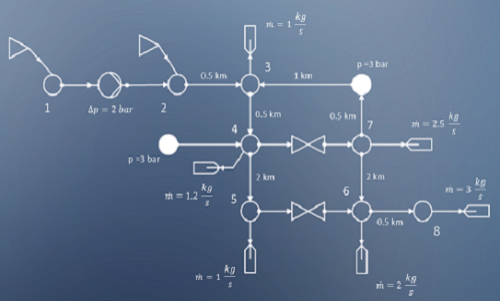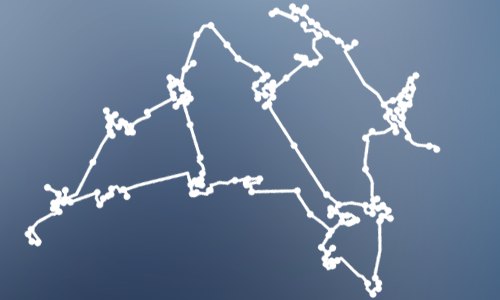To get started with pandapipes, just

1. Install pandapipes through pip:

`````` pip install pandapipes
``````
2. Create a simple network

`````` import pandapipes as pp
net = pp.create_empty_network(fluid="lgas")
j1 = pp.create_junction(net, pn_bar=1.05, tfluid_k=293.15, name="Junction 1")
j2 = pp.create_junction(net, pn_bar=1.05, tfluid_k=293.15, name="Junction 2")
j3 = pp.create_junction(net, pn_bar=1.05, tfluid_k=293.15, name="Junction 3")
ext_grid = pp.create_ext_grid(net, junction=j1, p_bar=1.1, t_k=293.15, name="Grid Connection")
sink = pp.create_sink(net, junction=j3, mdot_kg_per_s=0.045, name="Sink")
pipe = pp.create_pipe_from_parameters(net, from_junction=j1, to_junction=j2, length_km=0.1, diameter_m=0.05, name="Pipe 1")
valve = pp.create_valve(net, from_junction=j2, to_junction=j3, diameter_m=0.05, opened=True, name="Valve 1")
``````
3. Run a pipe flow:

`````` pp.pipeflow(net)
``````
4. And check the results:

`````` print(net.res_junction)
print(net.res_pipe)
``````

But of course pandapipes can do much more than that - find out what on this page!## Fluid Modeling

Includes thoroughly validated models for pipes, pumps, valves and more.## Fluid System Analysis

Supports stationary and quasi-stationary analysis of gas and district heating networks.## Free and Open

Published under a BSD License and therefore free to use, modify and share however you want.